﻿ 基于逐步回归的区域综合环境治理决策分析——以江苏省为例 Decision Analysis of Regional Comprehensive Environmental Governance Based on Stepwise Regression —Take Jiangsu Province as an Example

Computer Science and Application
Vol. 09  No. 09 ( 2019 ), Article ID: 32050 , 12 pages
10.12677/CSA.2019.99184

Decision Analysis of Regional Comprehensive Environmental Governance Based on Stepwise Regression —Take Jiangsu Province as an Example

Zhengyang Cao1, Peng Liu2, Aling Song1, Haifeng Liu1*

1Basic Department, Army Engineering University of PLA, Nanjing Jiangsu

2School of Automation, Southeast University, Nanjing Jiangsu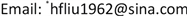Received: Aug. 14th, 2019; accepted: Aug. 29th, 2019; published: Sep. 5th, 2019ABSTRACT

The study on the relationship between economic development and environmental governance is of great theoretical significance and practical demand. In this paper, based on the weight of the entropy weight method, the stepwise linear regression method is used to construct the evaluation model of the relationship between economic development, environmental protection investment, and environmental pollution. Furthermore, this paper focuses on 18 indexes relating to these three factors to quantitatively evaluate the relationship between them. These conclusions are drawn from relevant data obtained from Jiangsu Province over the past 21 years. Likewise, this paper also evaluates the linear expression of these three indexes, and the influence on economic development is therefore also obtained. Finally, on the basis of verifying the effectiveness of the model, this paper puts forth several suggestions on the relationship between economic development and environmental governance.

Keywords:Stepwise Linear Regression, Economic Development, Comprehensive Ecological Improvement, Industrial Sewage Discharge, Environmental Protection Investment

1解放军陆军工程大学基础部，江苏 南京

2东南大学自动化学院，江苏 南京1. 引言

2. 基于逐步线性回归的经济发展综合评估模型构建及应用

2.1. 线性回归分析数据统计模型构建

$y={\beta }_{0}+{\beta }_{1}{x}_{1}+{\beta }_{2}{x}_{2}+\cdots +{\beta }_{n}{x}_{n}+\epsilon$

F检验。提出假设 ${H}_{0}:{\beta }_{1}={\beta }_{2}=\cdots ={\beta }_{k}=0$ ，(其中至少有一个 ${\beta }_{j}\ne 0$ )

${H}_{1}$ ：至少有一个  不等于0，${H}_{1}:{\beta }_{1}\ne 0$

${H}_{0}:{\beta }_{1}=0$

${H}_{1}:{\beta }_{1}\ne 0$

$t\sim t\left(n-k-1\right)$

2.2. 江苏省经济发展及环保投资数据统计

2.2.1. 数据来源及处理

2.2.2. 江苏省经济与环保数据概况统计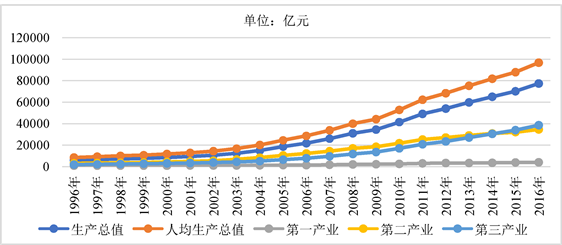Figure 1. Financial data of Jiangsu province 1996~2016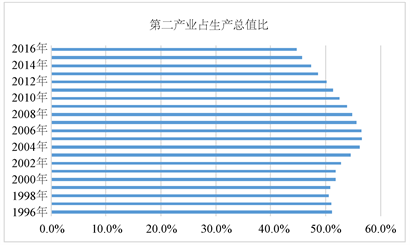Figure 2. The proportion of secondary industry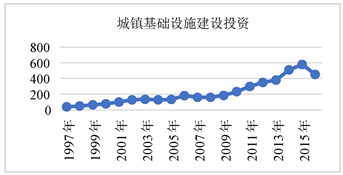Figure 3. The investment of infrastructure construction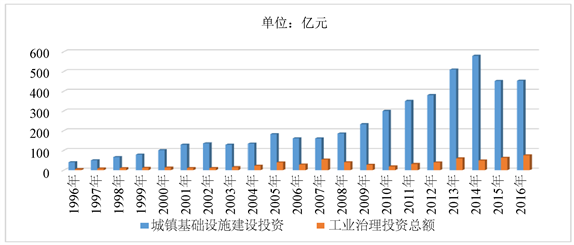Figure 4. The investment of infrastructure construction and Industrial governance

3. 经济发展、环保投资以及工业污染之关系评估——以江苏省为例

3.1. 经济发展与环境治理关系评估指标选取

3.2. 数据预处理

${Y}_{1}=0.193{x}_{1}+0.217{x}_{2}+0.202{x}_{3}+0.207{x}_{4}+0.181{x}_{5}$ (3.1)

${Y}_{2}=0.136{z}_{1}+0.146{z}_{2}+0.129{z}_{3}+0.126{z}_{4}+0.129{z}_{5}+0.119{z}_{6}+0.13{z}_{7}+0.128{z}_{8}$ (3.2)

${Y}_{3}=0.201{w}_{1}+0.201{w}_{2}+0.197{w}_{3}+0.204{w}_{4}+0.197{w}_{5}$ (3.3)Table 2. Synthetical financial data of Jiangsu province 1996~2016

4. 基于多元逐步线性回归的江苏省经济发展环保治理关系分析

4.1. 模型设计及实验结果分析Table 3. Data of synthetically financial and industrial governance 1996-2016

${Y}_{3}=-0.098{x}_{4}+0.46{x}_{5}+0.537{z}_{7}$ (4.1)

4.2. 模型设计有效性验证分析Table 7. Collinearity coefficient of evaluation indicator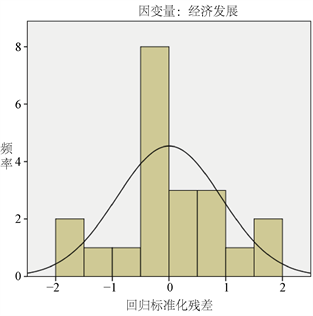Figure 5. The graph of residuals

5. 基于模型评估的结论与政策建议

Decision Analysis of Regional Comprehensive Environmental Governance Based on Stepwise Regression —Take Jiangsu Province as an Example[J]. 计算机科学与应用, 2019, 09(09): 1643-1654. https://doi.org/10.12677/CSA.2019.99184

1. 1. 符淼, 黄灼明. 我国经济发展阶段和环境污染的库兹涅茨关系[J]. 中国工业经济, 2008(6): 35-43.

2. 2. Ekins, P. (1997) The Kuznets Curve for the Environment and Economic Growth: Examining the Evidence. Environment and Plan-ning, 29, 805-829. https://doi.org/10.1068/a290805

3. 3. Schou, P. (2000) Polluting Non-Renewable Resources and Growth. Environmental and Resource Economics, 16, 211-227.

4. 4. Quah, E. and Boon, T.L. (2003) The Econom-ic Cost of Particulate Air Pollution on Health in Singapore. Journal of Asian Economics, 14, 73-90. https://doi.org/10.1016/S1049-0078(02)00240-3

5. 5. 邵海清. 环保投资与国民经济增长的灰色关联分析[J]. 生产力研究, 2010(12): 14-15.

6. 6. 雷社平, 何音音. 我国环保投资与经济增长的回归分析[J]. 西北工业大学学报(社会科学版), 2010, 30(2): 20-22.

7. 7. 游士兵, 严研. 逐步回归分析法及其应用[J]. 统计与决策, 2017(14): 31-35.

8. 8. 冯智涛, 张瑜, 姚靓, 宋雅婷, 潘晶. 县域经济评价指标的赋权方法研究——以京津冀城市群为例[J]. 科技经济市场, 2018(9): 52-56.

9. 9. 钟志威. 环境污染与经济发展的关系[D]: [硕士学位论文]. 广州: 暨南大学, 2010.

10. 10. 夏庆澍. 中国经济增长与环境污染关系的实证性研究[D]: [硕士学位论文]. 昆明: 云南财经大学, 2011.

11. NOTES

*通讯作者。